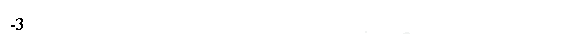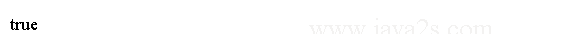# Java Object Oriented Design - Java Enum Compare

You can compare two enum constants in three ways:

• Using the compareTo() method of the Enum class
• Using the equals() method of the Enum class
• Using the == operator

The compareTo() method of the Enum class compares two enum constants of the same enum type. It returns the difference in ordinal for the two enum constants. If both enum constants are the same, it returns zero.

## Example

The following code will print -3 because the difference of the ordinals for LOW(ordinal=0) and URGENT(ordinal=3) is -3.

A negative value means the constant being compared occurs before the one being compared against.

```enum Level {/*from  w  w w .java  2  s . c  o m*/
LOW, MEDIUM, HIGH, URGENT;
}

public class Main {
public static void main(String[] args) {
Level s1 = Level.LOW;
Level s2 = Level.URGENT;

// s1.compareTo(s2) returns s1.ordinal() - s2.ordinal()
int diff = s1.compareTo(s2);
System.out.println(diff);
}
}
```

The code above generates the following result.## Example 2

The equals() method of the Enum class compares two enum constants for equality.

An enum constant is equal only to itself. The equals() method can be invoked on two enum constants of different types.

```enum Level {//w  w  w .  j av  a  2  s .  c  om
LOW, MEDIUM, HIGH, URGENT;
}

public class Main {
public static void main(String[] args) {
Level s1  = Level.LOW;
Level s2  = Level.URGENT;
System.out.println(s1.equals(s1));
}
}
```

The code above generates the following result.We can use the equality operator `==` to compare two enum constants for equality.

Both operands to the == operator must be of the same enum type.